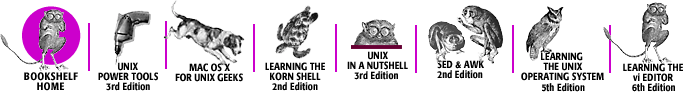home | O'Reilly's CD bookshelfs | FreeBSD | Linux | Cisco | Cisco Exam## 12.9. Special Characters

This section lists the following special characters:

• Characters that reside on the standard fonts

• Miscellaneous characters

• Bracket-building symbols

• Mathematics symbols

• Greek characters

The characters in the first table below are available on the standard fonts. The characters in the remaining tables are available only on the special font.

#### Table 12-2. Characters on the Standard Fonts

InputCharCharacter Name
''Close quote
Open quote
\(em--Em-dash (width of “m”)
\(enEn-dash (width of “n”)
\--Minus in current font
--Hyphen
\(hy-Hyphen
\(bu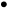Bullet
\(sq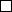Square
\(ru_Rule
\(14¼1/4
\(12½1/2
\(34¾3/4
\(fi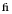fi ligature
\(fl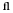fl ligature
\(ff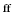ff ligature
\(Fi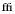ffi ligature
\(Fl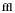ffl ligature
\(de°Degree
\(dg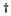Dagger
\(fm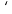Foot mark
\(ct¢Cent sign
\(rg®Registered

#### Table 12-3. Miscellaneous Characters

InputCharCharacter Name
\(sc§Section
\(aa´Acute accent
´Acute accent
\(ga`Grave accent
\``Grave accent
\(ul_Underrule
\(->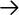Right arrow
\(<-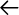Left arrow
\(ua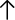Up arrow
\(da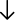Down arrow
\(br|Box rule
\(ddDouble dagger
\(rh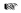Right hand
\(lh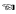Left hand
\(ci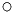Circle

#### Table 12-4. Bracket-Building Symbols

InputCharCharacter Name
\(lt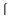Left top of big curly bracket
\(lk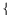Left center of big curly bracket
\(lb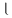Left bottom of big curly bracket
\(rt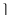Right top of big curly bracket
\(rk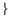Right center of big curly bracket
\(rb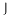Right bottom of big curly bracket
\(lc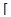Left ceiling (left top) of big square bracket
\(bv|Bold vertical
\(lf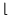Left floor (left bottom) of big square bracket
\(rc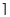Right ceiling (right top) of big square bracket
\(rf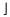Right floor (right bottom) of big square bracket

#### Table 12-4. Mathematics Symbols

InputCharCharacter Name
\(pl+Math plus
\(mi-Math minus
\(eq=Math equals
\(***Math star
\(sl/Slash (matching backslash)
\(sr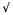Square root
\(rn-Root en extender
\(>=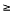Greater than or equal to
\(<=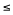Less than or equal to
\(==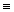Identically equal
\(~~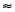Approximately equal
\(ap~Approximates
\(!=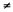Not equal
\(mu×Multiply
\(di÷Divide
\(+-±Plus-minus
\(cu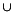Cup (union)
\(ca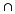Cap (intersection)
\(sb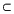Subset of
\(sp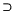Superset of
\(ib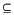Improper subset
\(ip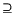Improper superset
\(if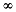Infinity
\(pd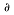Partial derivative
\(gr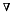Gradient
\(no¬Not
\(is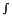Integral sign
\(pt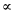Proportional to
\(es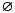Empty set
\(mo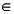Member of
\(or|Or